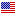1st Edition

# Complex Networks An Algorithmic Perspective

ISBN 9781138033894
320 Pages 184 B/W Illustrations
Published October 12, 2017 by CRC PressFree Shipping (6-12 Business Days)
shipping options

Original Price \$62.95
Sale Price USD \$50.36
ISBN 9781466571662
320 Pages 184 B/W Illustrations
Published September 6, 2014 by CRC PressFree Shipping (6-12 Business Days)
shipping options

Original Price \$200.00
Sale Price USD \$160.00
ISBN 9780429072031
320 Pages
Published September 6, 2014 by CRC Press

Also available as eBook on:

Network science is a rapidly emerging field of study that encompasses mathematics, computer science, physics, and engineering. A key issue in the study of complex networks is to understand the collective behavior of the various elements of these networks.

Although the results from graph theory have proven to be powerful in investigating the structures of complex networks, few books focus on the algorithmic aspects of complex network analysis. Filling this need, Complex Networks: An Algorithmic Perspective supplies the basic theoretical algorithmic and graph theoretic knowledge needed by every researcher and student of complex networks.

This book is about specifying, classifying, designing, and implementing mostly sequential and also parallel and distributed algorithms that can be used to analyze the static properties of complex networks. Providing a focused scope which consists of graph theory and algorithms for complex networks, the book identifies and describes a repertoire of algorithms that may be useful for any complex network.

• Provides the basic background in terms of graph theory
• Supplies a survey of the key algorithms for the analysis of complex networks
• Presents case studies of complex networks that illustrate the implementation of algorithms in real-world networks, including protein interaction networks, social networks, and computer networks

Requiring only a basic discrete mathematics and algorithms background, the book supplies guidance that is accessible to beginning researchers and students with little background in complex networks. To help beginners in the field, most of the algorithms are provided in ready-to-be-executed form.

While not a primary textbook, the author has included pedagogical features such as learning objectives, end-of-chapter summaries, and review questions

BACKGROUND

Introduction
Overview
Real-World Complex Networks
Technological Networks
Information Networks
Social Networks
Biological Networks
Topological Properties of Complex Networks
Algorithmic Challenges
Outline of the Book
References

Graph Theory
Basics
Subgraphs
Graph Isomorphism
Types of Graphs
Paths and Cycles
Connectivity
Trees
Graph Representations
Spectral Properties of Graphs
Eigenvalues and Eigenvectors
The Laplacian Matrix
Chapter Notes
References

Algorithms and Complexity
Introduction
Time Complexity
Recurrences
Divide and Conquer Algorithms
Graph Algorithms
Depth-first Search
Dynamic Programming
Greedy Algorithms
NP-Complete Problems
NP Completeness
Reductions
Satisfiability Problems
3-SAT to Independent Set
Independent Set to Vertex Cover
Independent Set to Clique
Coping with NP Completeness
Backtracking
Branch and Bound
Approximation Algorithms
Parallel Algorithms
Architectural Constraints
Example Algorithms
Distributed Systems and Algorithms
Chapter Notes
References

Analysis of Complex Networks
Introduction
Vertex Degrees
Degree Sequence
Degree Distribution
Communities
Clustering Coefficient
The Matching Index
Centrality
Network Motifs
Models
Small World Networks
Scale-Free Networks
Chapter Notes
References

ALGORITHMS

Distance and Centrality
Introduction
Finding Distances
Average Distance
Dijkstra’s Single Source Shortest Paths Algorithm
Floyd-Warshall All Pairs Shortest Paths Algorithm
Centrality
Degree Centrality
A Distributed Algorithm for k-hop Degree Centrality
Closeness Centrality
Stress Centrality
Betweenness Centrality
Newman’s Algorithm
Brandes’ Algorithm
Eigenvalue Centrality
Chapter Notes
References

Special Subgraphs
Introduction
Maximal Independent Sets
Dominating Sets
A Greedy MDS Algorithm
Guha-Khuller First MCDS Algorithm
Guha-Khuller Second MCDS Algorithm
Matching
A Maximal Unweighted Matching Algorithm
A MaximalWeighted Matching Algorithm
Vertex Cover
A Minimal Connected Vertex Cover Algorithm
A Minimal Weighted Vertex Cover Algorithm
A Distributed Algorithm for MWVC Construction
Chapter Notes
References

Data Clustering
Introduction
Types of Data Clustering
Agglomerative Hierarchical Clustering
k-means Algorithm
Nearest Neighbor Algorithm
Fuzzy Clustering
Density-based Clustering
Parallel Data Clustering
Chapter Notes
References

Graph-based Clustering
Introduction
Graph Partitioning
BFS-based Partitioning
Kernighan-Lin Algorithm
Spectral Bisection
Multi-level Partitioning
Parallel Partitioning
Graph Clustering
MST-based Clustering
Discovery of Dense Subgraphs
Definitions
Clique Algorithms
The First Algorithm
The Second Algorithm
k-core Algorithm
Chapter Notes
References

Network Motif Discovery
Introduction
Network Motifs
Measures of Motif Significance
Generating Null Models
Hardness of Motif Discovery
Subgraph Isomorphism
Vertex Invariants
Algorithms
Ullman’s Algorithm
Nauty Algorithm
VF2 Algorithm
BM1 Algorithm
Motif Discovery Algorithms
Exact Census Algorithms
Mf inder Algorithm
Enumerate Subgraphs (ESU) Algorithm
Grochow and Kellis Algorithm
Kavosh Algorithm
MODA
Approximate Algorithms with Sampling
Mf inder with Sampling
Randomized ESU Algorithm
MODA with Sampling
Chapter Notes
References

APPLICATIONS

Protein Interaction Networks
Introduction
Topological Properties of PPI Networks
Detection of Protein Complexes
Highly Connected Subgraphs Algorithm
Restricted Neighborhood Search Algorithm
Molecular Complex Detection Algorithm
Markov Clustering Algorithm
Network Motifs in PPI Networks
Network Alignment
Quality of the Alignment
Topological Similarity
Node Similarity
Algorithms
PathBLAST
MaWIsh
IsoRank
GRAAL
Recent Algorithms
Chapter Notes
References

Social Networks

Introduction
Relationships
Homophily
Positive and Negative Relations
Structural Balance
Equivalence
Community Detection Algorithms
Edge Betweenness-based Algorithm
Resistor Networks
RandomWalk Centrality
Modularity-based Algorithm
Chapter Notes
References

The Internet and the Web
Introduction
The Internet
Services
Services of Connection
Circuit and Packet Switching
Internet Protocol Suite
Analysis
The Web
The Web Graph
Properties
Models
Evolving Model
Copying Model
Growth-deletion Model
Multi-layer Model
Cyber Community Detection
Hubs and Authorities
Page Rank Algorithm
Chapter Notes
References

Introduction
Clustering Algorithms
Lowest-ID Algorithm
Dominating Set-based Clustering
Spanning Tree-based Clustering
Mobile Social Networks
Architecture
Community Detection
Middleware
MobiSoC
MobiClique
SAMOA
Yarta
Chapter Notes
References
Index

### Biography

Kayhan Erciyes is a professor of computer science and engineering and also the rector of Izmir University, Izmir, Turkey. Dr. Erciyes worked as a research and development engineer of Alcatel Turkey, Alcatel Portugal, and Alcatel SEL. He has worked as faculty in Oregon State University, UC Davis and California State University, US and Izmir and Aegean universities. His research interests are on distributed systems, graph theory and distributed algorithms for complex networks, mobile ad hoc networks, wireless sensor networks and the Grid and has published extensively in these areas. Dr. Erciyes is the designer and implementer of one of the first commercially available MODEMs in Turkey

We offer free standard shipping on every order across the globe.